# C hapter 3 Recursion Chapter 3 Recursion 3

• Slides: 64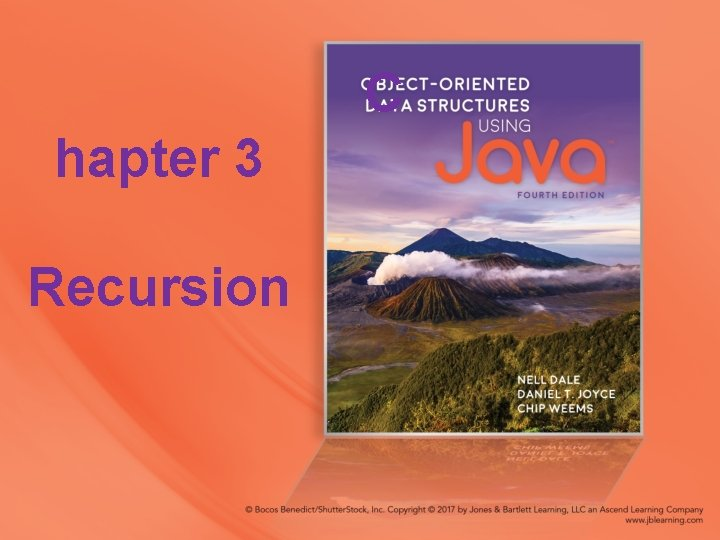C hapter 3 Recursion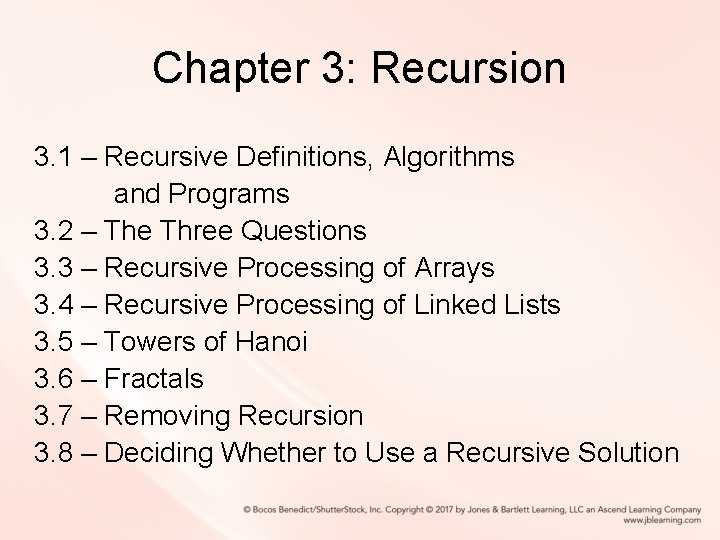Chapter 3: Recursion 3. 1 – Recursive Definitions, Algorithms and Programs 3. 2 – The Three Questions 3. 3 – Recursive Processing of Arrays 3. 4 – Recursive Processing of Linked Lists 3. 5 – Towers of Hanoi 3. 6 – Fractals 3. 7 – Removing Recursion 3. 8 – Deciding Whether to Use a Recursive Solution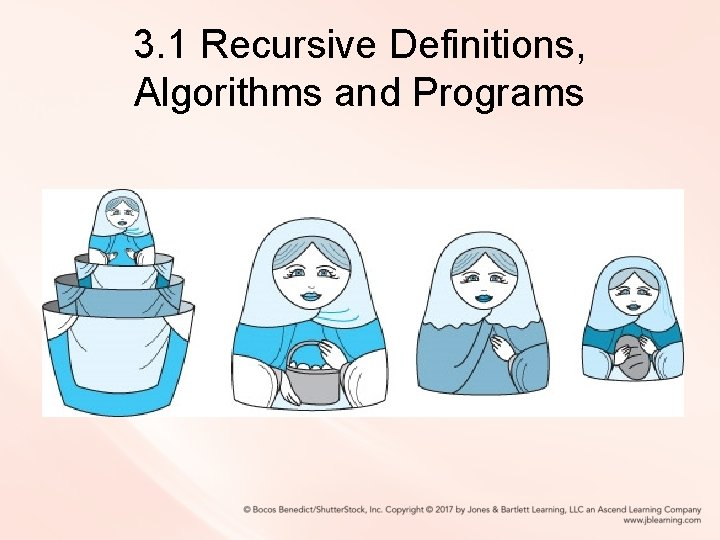3. 1 Recursive Definitions, Algorithms and Programs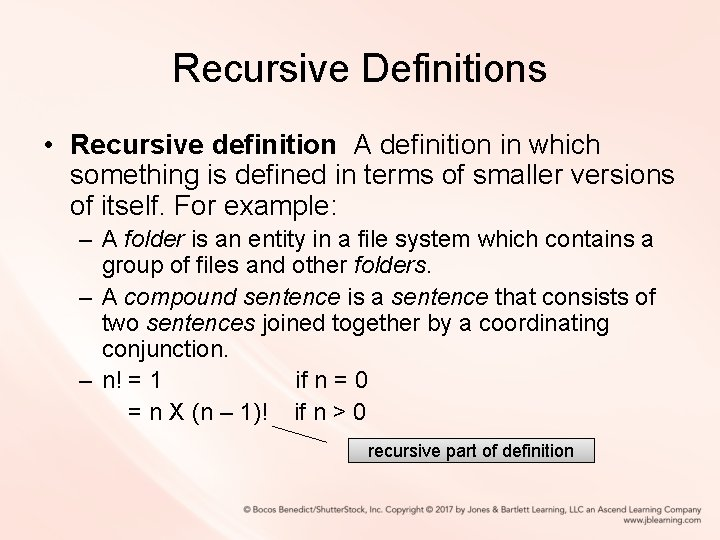Recursive Definitions • Recursive definition A definition in which something is defined in terms of smaller versions of itself. For example: – A folder is an entity in a file system which contains a group of files and other folders. – A compound sentence is a sentence that consists of two sentences joined together by a coordinating conjunction. – n! = 1 if n = 0 = n X (n – 1)! if n > 0 recursive part of definition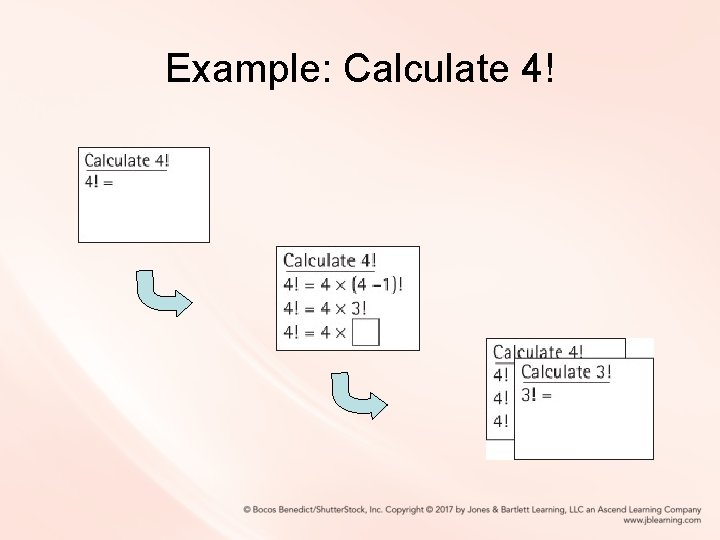Example: Calculate 4!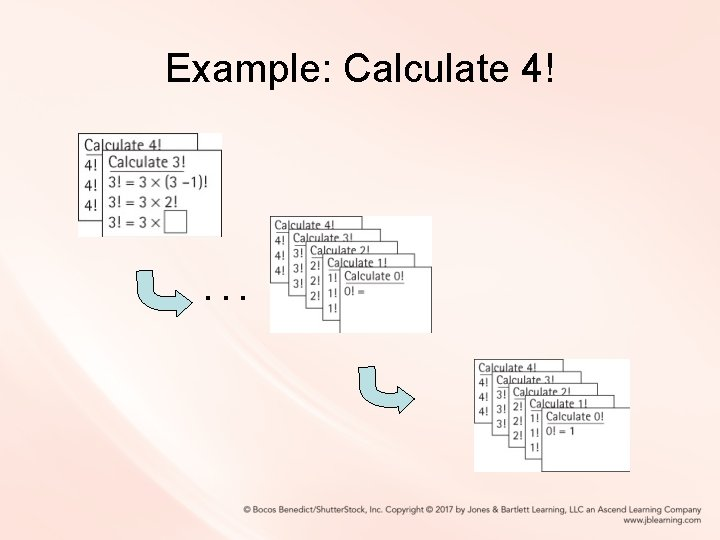Example: Calculate 4! . . .Example: Calculate 4! . . .Recursive Algorithms • Recursive algorithm A solution that is expressed in terms of – smaller instances of itself and – a base case • Base case The case for which the solution can be stated non-recursively • General (recursive) case The case for which the solution is expressed in terms of a smaller version of itselfExamples of a Recursive Algorithm and a Recursive Program Factorial (int n) // Assume n >= 0 if (n == 0) return (1) else return ( n * Factorial ( n – 1 ) ) public static int factorial(int n) // Precondition: n is non-negative // // Returns the value of "n!". { if (n == 0) return 1; // Base case else return (n * factorial(n – 1)); } // General caseRecursion Terms • Recursive call A method call in which the method being called is the same as the one making the call • Direct recursion Recursion in which a method directly calls itself, like the factorial method. • Indirect recursion Recursion in which a chain of two or more method calls returns to the method that originated the chain, for example method A calls method B which in turn calls method AIterative Solution for Factorial • We have used the factorial algorithm to demonstrate recursion because it is familiar and easy to visualize. In practice, one would never want to solve this problem using recursion, since a straightforward, more efficient iterative solution exists: public static int factorial(int n) { int ret. Value = 1; while (n != 0) { ret. Value = ret. Value * n; n = n - 1; } return(ret. Value); }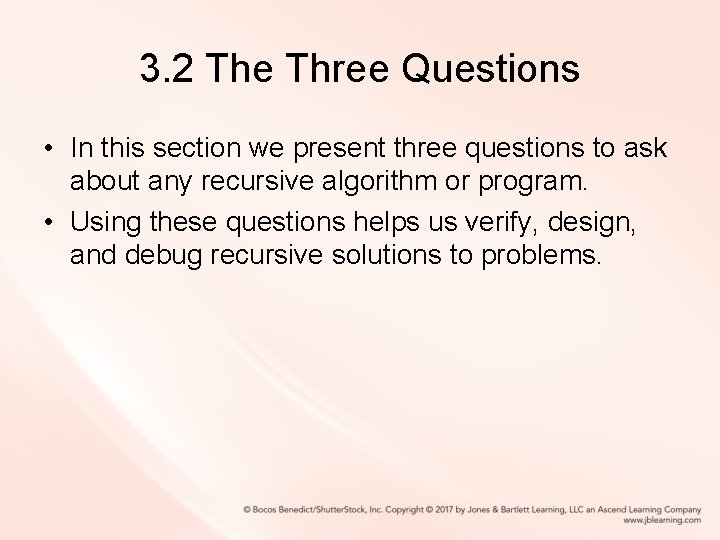3. 2 The Three Questions • In this section we present three questions to ask about any recursive algorithm or program. • Using these questions helps us verify, design, and debug recursive solutions to problems.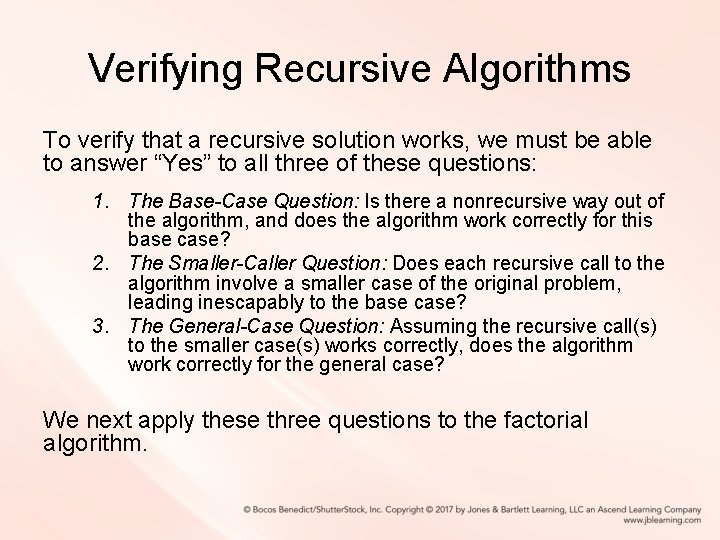Verifying Recursive Algorithms To verify that a recursive solution works, we must be able to answer “Yes” to all three of these questions: 1. The Base-Case Question: Is there a nonrecursive way out of the algorithm, and does the algorithm work correctly for this base case? 2. The Smaller-Caller Question: Does each recursive call to the algorithm involve a smaller case of the original problem, leading inescapably to the base case? 3. The General-Case Question: Assuming the recursive call(s) to the smaller case(s) works correctly, does the algorithm work correctly for the general case? We next apply these three questions to the factorial algorithm.The Base-Case Question Factorial (int n) // Assume n >= 0 if (n == 0) return (1) else return ( n * Factorial ( n – 1 ) ) Is there a nonrecursive way out of the algorithm, and does the algorithm work correctly for this base case? The base case occurs when n is 0. The Factorial algorithm then returns the value of 1, which is the correct value of 0!, and no further (recursive) calls to Factorial are made. The answer is yes.The Smaller-Caller Question Factorial (int n) // Assume n >= 0 if (n == 0) return (1) else return ( n * Factorial ( n – 1 ) ) Does each recursive call to the algorithm involve a smaller case of the original problem, leading inescapably to the base case? The parameter is n and the recursive call passes the argument n - 1. Therefore each subsequent recursive call sends a smaller value, until the value sent is finally 0. At this point, as we verified with the base-case question, we have reached the smallest case, and no further recursive calls are made. The answer is yes.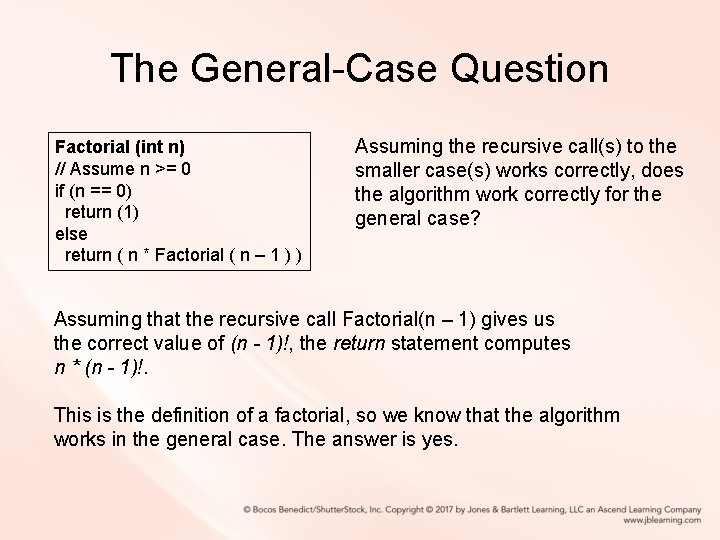The General-Case Question Factorial (int n) // Assume n >= 0 if (n == 0) return (1) else return ( n * Factorial ( n – 1 ) ) Assuming the recursive call(s) to the smaller case(s) works correctly, does the algorithm work correctly for the general case? Assuming that the recursive call Factorial(n – 1) gives us the correct value of (n - 1)!, the return statement computes n * (n - 1)!. This is the definition of a factorial, so we know that the algorithm works in the general case. The answer is yes.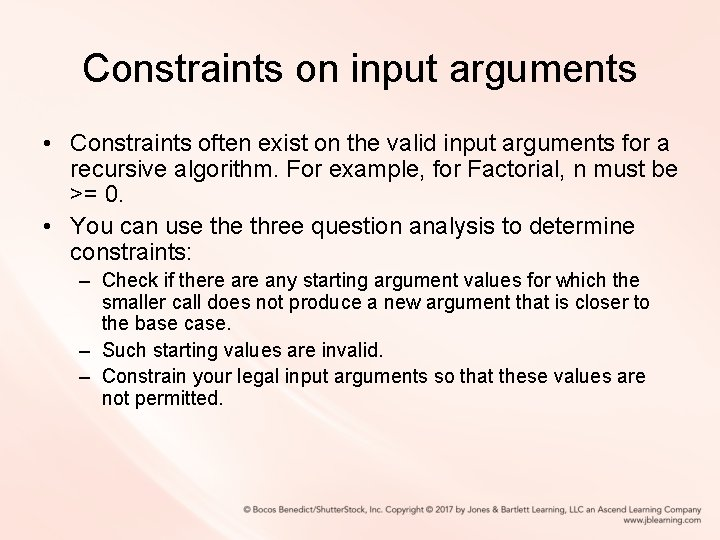Constraints on input arguments • Constraints often exist on the valid input arguments for a recursive algorithm. For example, for Factorial, n must be >= 0. • You can use three question analysis to determine constraints: – Check if there any starting argument values for which the smaller call does not produce a new argument that is closer to the base case. – Such starting values are invalid. – Constrain your legal input arguments so that these values are not permitted.Steps for Designing Recursive Solutions 1. Get an exact definition of the problem to be solved. 2. Determine the size of the problem to be solved on this call to the method. 3. Identify and solve the base case(s) in which the problem can be expressed non-recursively. This ensures a yes answer to the base-case question. 4. Identify and solve the general case(s) correctly in terms of a smaller case of the same problem—a recursive call. This ensures yes answers to the smaller-caller and general-case questions.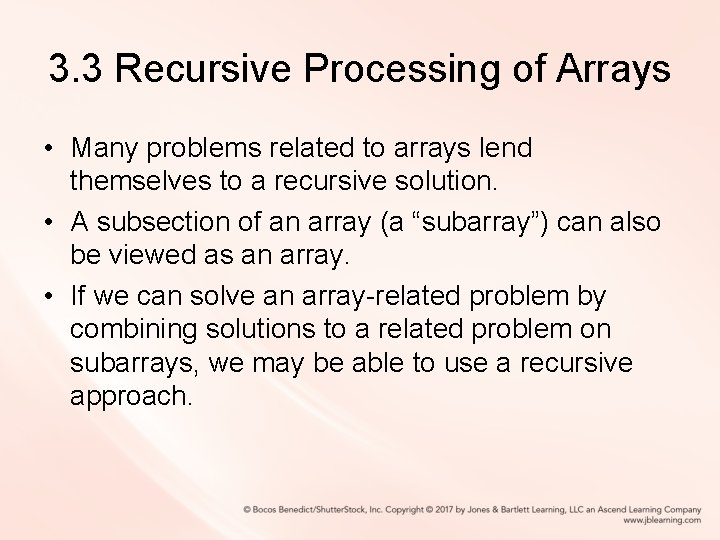3. 3 Recursive Processing of Arrays • Many problems related to arrays lend themselves to a recursive solution. • A subsection of an array (a “subarray”) can also be viewed as an array. • If we can solve an array-related problem by combining solutions to a related problem on subarrays, we may be able to use a recursive approach.Binary Search • Problem: find a target element in a sorted array • Approach – examine the midpoint of the array and compare the element found there to our target element – eliminate half the array from further consideration – recursively repeat this approach on the remaining half of the array until we find the target or determine that it is not in the array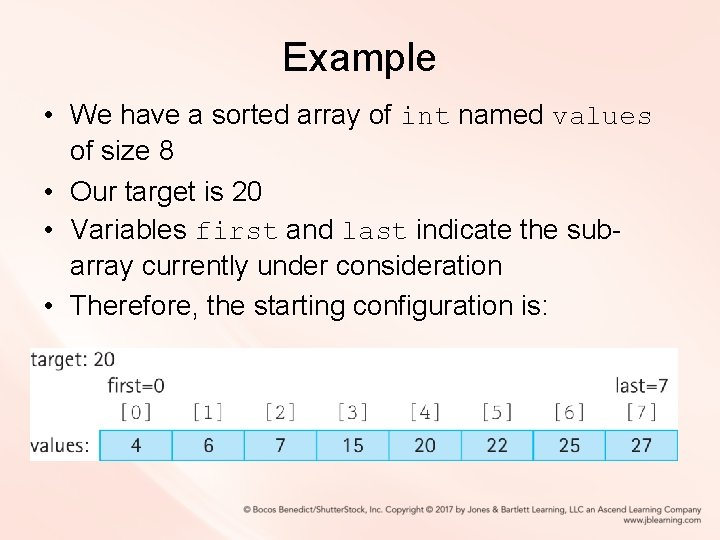Example • We have a sorted array of int named values of size 8 • Our target is 20 • Variables first and last indicate the subarray currently under consideration • Therefore, the starting configuration is: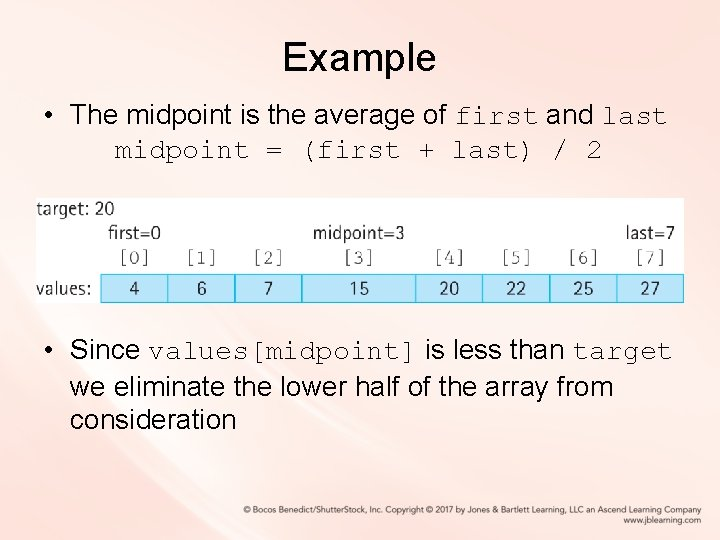Example • The midpoint is the average of first and last midpoint = (first + last) / 2 • Since values[midpoint] is less than target we eliminate the lower half of the array from consideration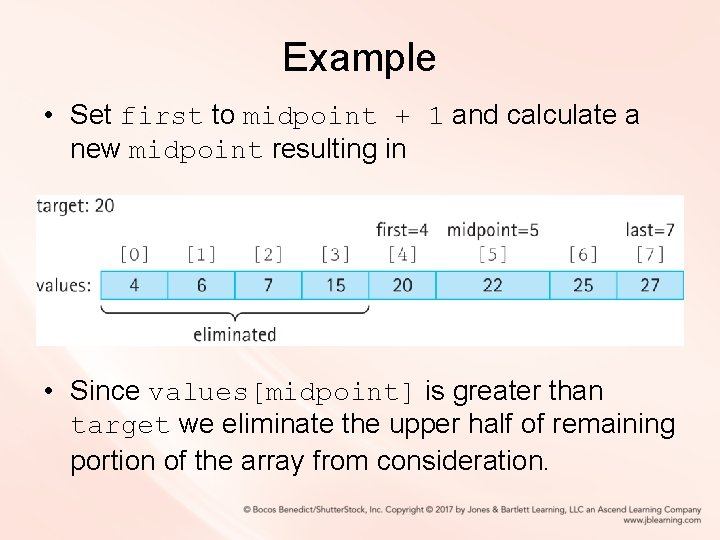Example • Set first to midpoint + 1 and calculate a new midpoint resulting in • Since values[midpoint] is greater than target we eliminate the upper half of remaining portion of the array from consideration.Example • Set last to midpoint - 1 and calculate a new midpoint resulting in • Since values[midpoint]equals target we are finished and return true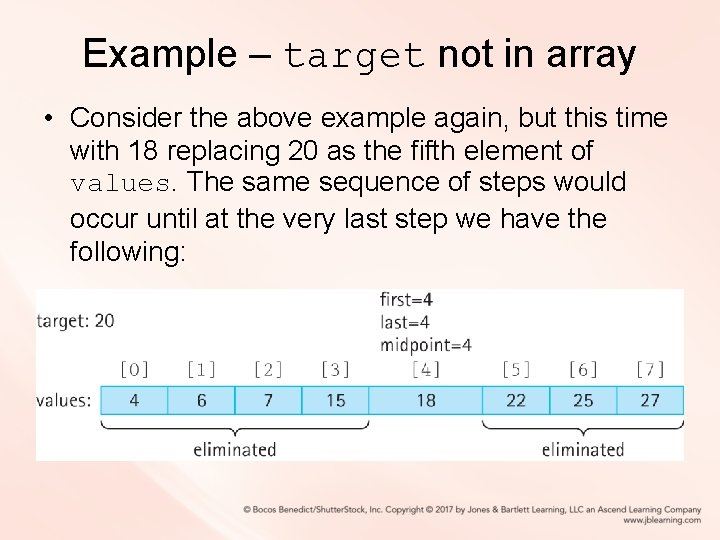Example – target not in array • Consider the above example again, but this time with 18 replacing 20 as the fifth element of values. The same sequence of steps would occur until at the very last step we have the following: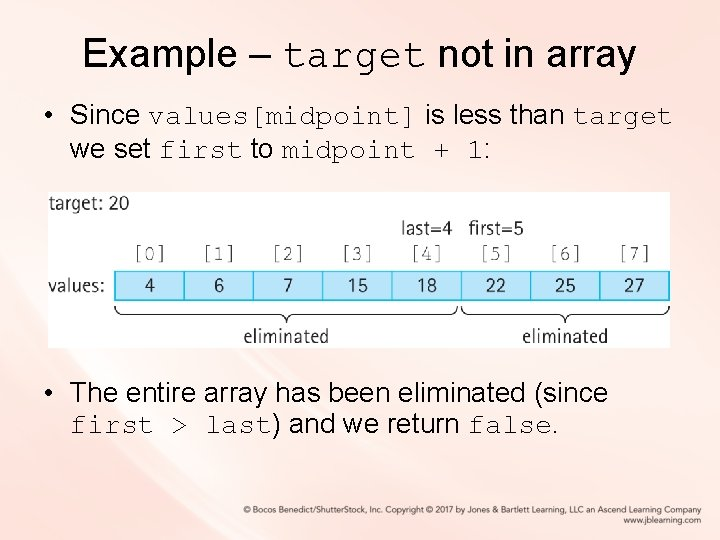Example – target not in array • Since values[midpoint] is less than target we set first to midpoint + 1: • The entire array has been eliminated (since first > last) and we return false.Code for the Binary Search boolean binary. Search(int target, int first, int last) // Precondition: first and last are legal indices of values // // If target is contained in values[first, last] return true // otherwise return false. { int midpoint = (first + last) / 2; if (first > last) return false; else if (target == values[midpoint]) return true; else if (target > values[midpoint]) return binary. Search(target, midpoint + 1, last); else return binary. Search(target, first, midpoint - 1); } recursive call3. 4 Recursive Processing of Linked Lists • A linked list is a recursive structure • The LLNode class, our building block for linked lists, is a self-referential (recursive) class: public class LLNode<T> { protected T info; // information stored in list protected LLNode<T> link; // reference to a node. . . recursive referenceRecursive nature of Linked Lists • A linked list is either empty or consists of a node containing two parts: – information – a linked list contains info A plus a linked list (B – C)Printing a Linked List Recursively void rec. Print. List(LLNode<String> list. Ref) { if (list. Ref != null) { System. out. println(list. Ref. get. Info()); rec. Print. List(list. Ref. get. Link()); } }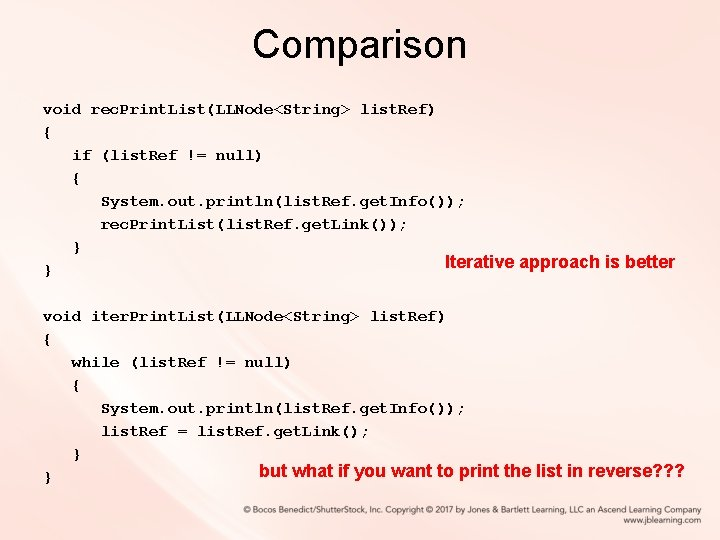Comparison void rec. Print. List(LLNode<String> list. Ref) { if (list. Ref != null) { System. out. println(list. Ref. get. Info()); rec. Print. List(list. Ref. get. Link()); } Iterative approach is better } void iter. Print. List(LLNode<String> list. Ref) { while (list. Ref != null) { System. out. println(list. Ref. get. Info()); list. Ref = list. Ref. get. Link(); } but what if you want to print the list in reverse? ? ? }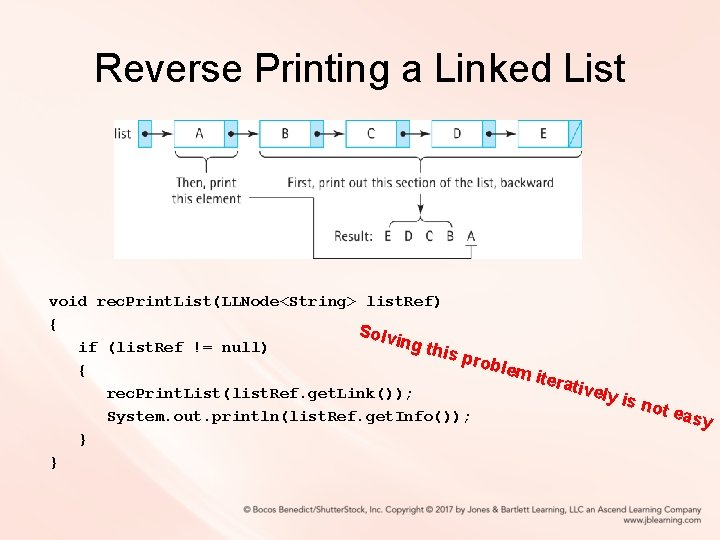Reverse Printing a Linked List void rec. Print. List(LLNode<String> list. Ref) { Solv ing t if (list. Ref != null) his p roble { m ite rativ ely is rec. Print. List(list. Ref. get. Link()); not easy System. out. println(list. Ref. get. Info()); } }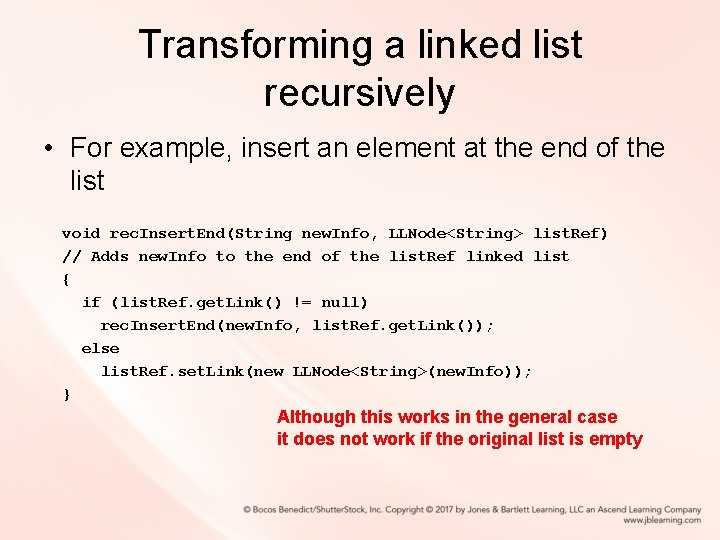Transforming a linked list recursively • For example, insert an element at the end of the list void rec. Insert. End(String new. Info, LLNode<String> list. Ref) // Adds new. Info to the end of the list. Ref linked list { if (list. Ref. get. Link() != null) rec. Insert. End(new. Info, list. Ref. get. Link()); else list. Ref. set. Link(new LLNode<String>(new. Info)); } Although this works in the general case it does not work if the original list is emptyProblem with the approach void rec. Insert. End(String new. Info, LLNode<String> list. Ref) // Adds new. Info to the end of the list. Ref linked list { if (list. Ref. get. Link() != null) rec. Insert. End(new. Info, list. Ref. get. Link()); else list. Ref. set. Link(new LLNode<String>(new. Info)); } If we invoke, for example, rec. Insert. End(“Z”, my. List) the temporary variable list. Ref will hold a linked list consisting of “Z” but my. List will still be null.Solution • Rather than return void where we invoke as rec. Insert. End(“Z”, my. List) • Need a solution that returns a linked list so we can invoke as my. List = rec. Insert. End(“Z”, my. List) • This is the only way we can change the value of my. List in the case that the list is empty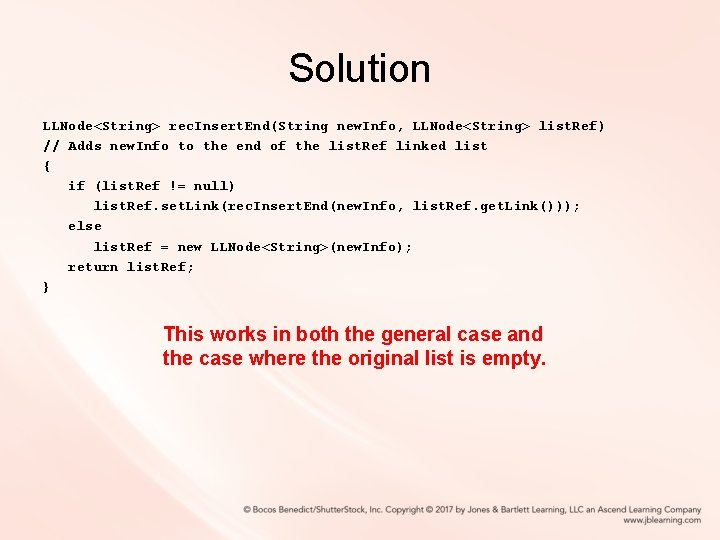Solution LLNode<String> rec. Insert. End(String new. Info, LLNode<String> list. Ref) // Adds new. Info to the end of the list. Ref linked list { if (list. Ref != null) list. Ref. set. Link(rec. Insert. End(new. Info, list. Ref. get. Link())); else list. Ref = new LLNode<String>(new. Info); return list. Ref; } This works in both the general case and the case where the original list is empty.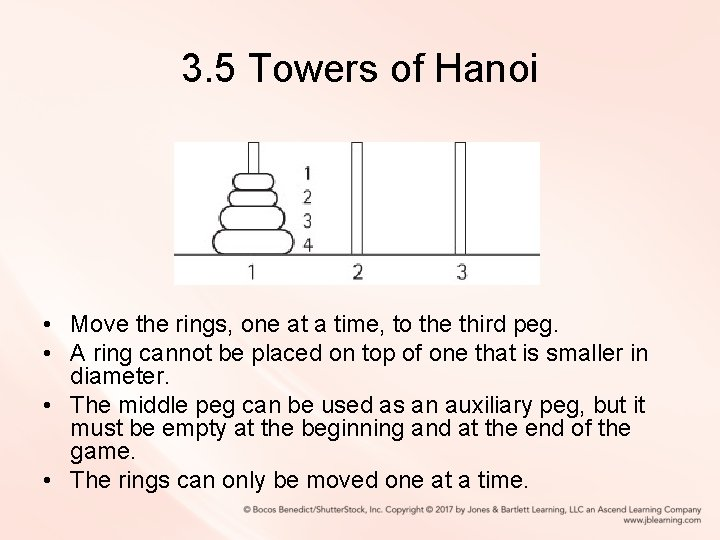3. 5 Towers of Hanoi • Move the rings, one at a time, to the third peg. • A ring cannot be placed on top of one that is smaller in diameter. • The middle peg can be used as an auxiliary peg, but it must be empty at the beginning and at the end of the game. • The rings can only be moved one at a time.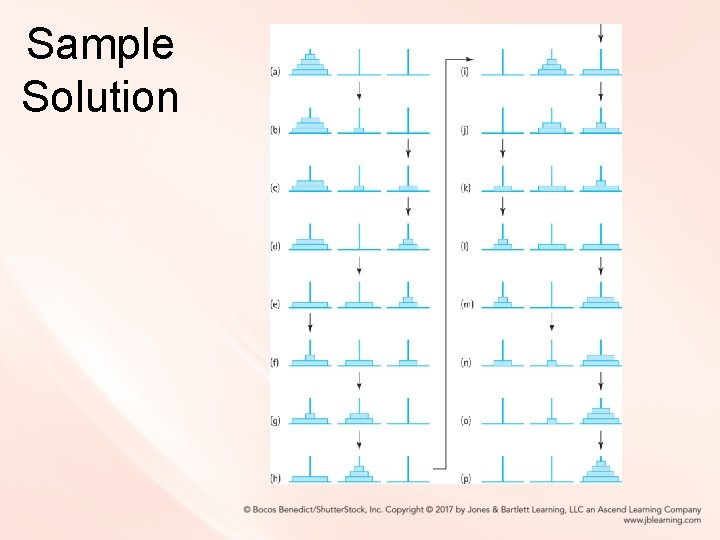Sample Solution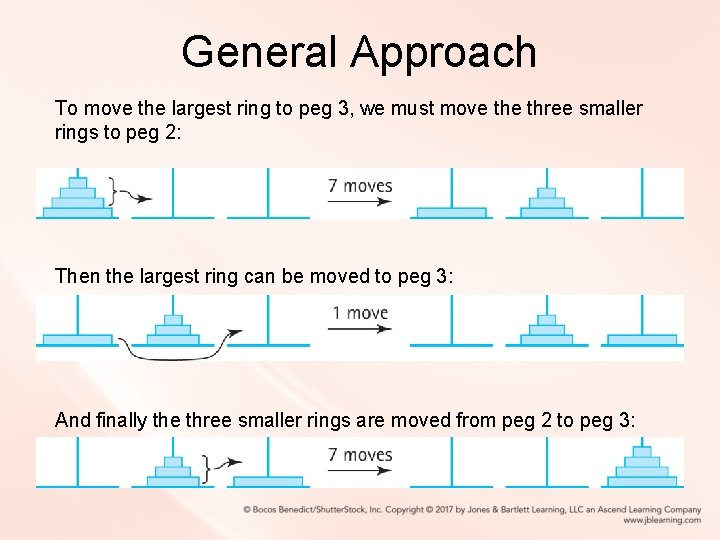General Approach To move the largest ring to peg 3, we must move three smaller rings to peg 2: Then the largest ring can be moved to peg 3: And finally the three smaller rings are moved from peg 2 to peg 3: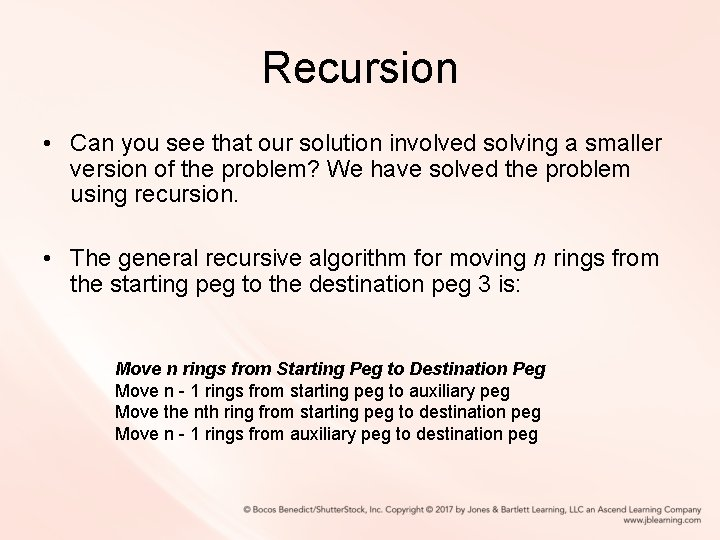Recursion • Can you see that our solution involved solving a smaller version of the problem? We have solved the problem using recursion. • The general recursive algorithm for moving n rings from the starting peg to the destination peg 3 is: Move n rings from Starting Peg to Destination Peg Move n - 1 rings from starting peg to auxiliary peg Move the nth ring from starting peg to destination peg Move n - 1 rings from auxiliary peg to destination peg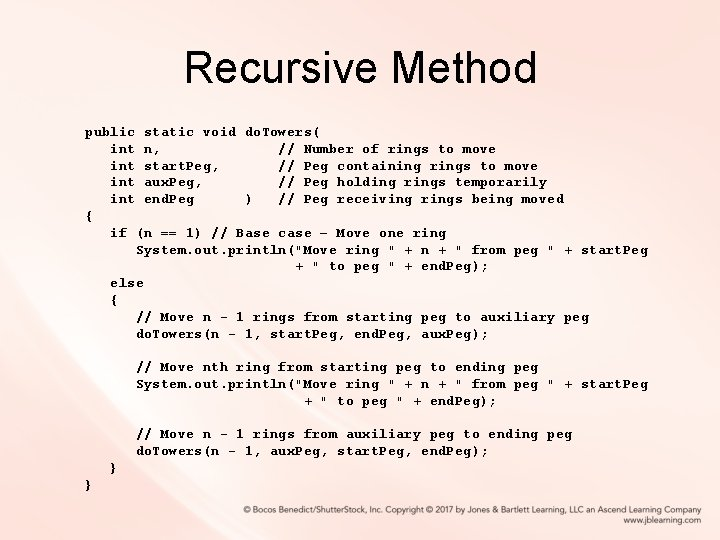Recursive Method public static void do. Towers( int n, // Number of rings to move int start. Peg, // Peg containing rings to move int aux. Peg, // Peg holding rings temporarily int end. Peg ) // Peg receiving rings being moved { if (n == 1) // Base case – Move one ring System. out. println("Move ring " + n + " from peg " + start. Peg + " to peg " + end. Peg); else { // Move n - 1 rings from starting peg to auxiliary peg do. Towers(n - 1, start. Peg, end. Peg, aux. Peg); // Move nth ring from starting peg to ending peg System. out. println("Move ring " + n + " from peg " + start. Peg + " to peg " + end. Peg); // Move n - 1 rings from auxiliary peg to ending peg do. Towers(n - 1, aux. Peg, start. Peg, end. Peg); } }Code and Demo • Instructors can now walk through the code contained in Towers. java in the ch 03. apps package and demonstrate the running program.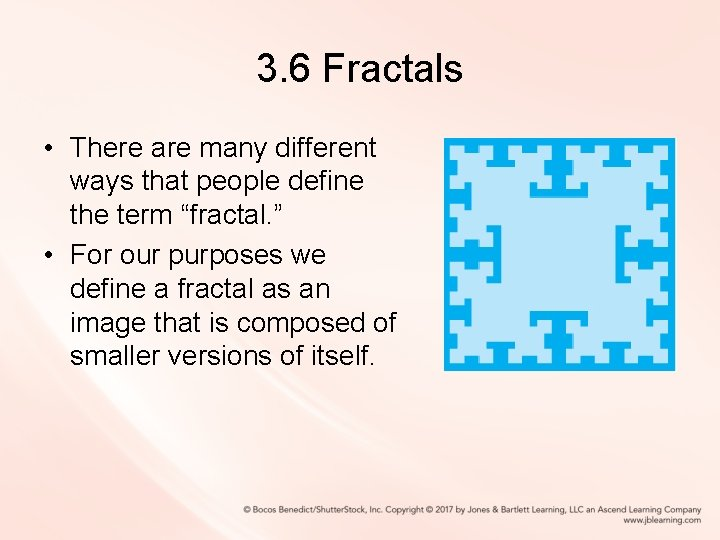3. 6 Fractals • There are many different ways that people define the term “fractal. ” • For our purposes we define a fractal as an image that is composed of smaller versions of itself.A T-Square Fractal • In the center of a square black* canvas we draw a white* square, one-quarter the size of the canvas: *Although our publisher supplied figures are tinted blue, our code generates black and white images.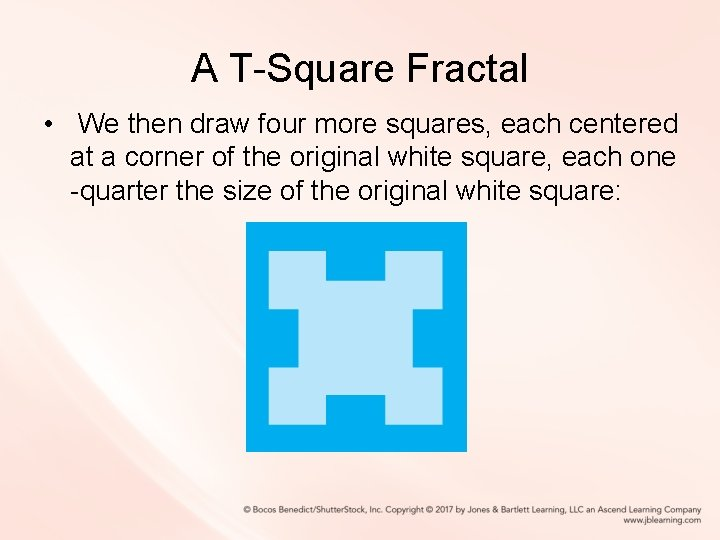A T-Square Fractal • We then draw four more squares, each centered at a corner of the original white square, each one -quarter the size of the original white square: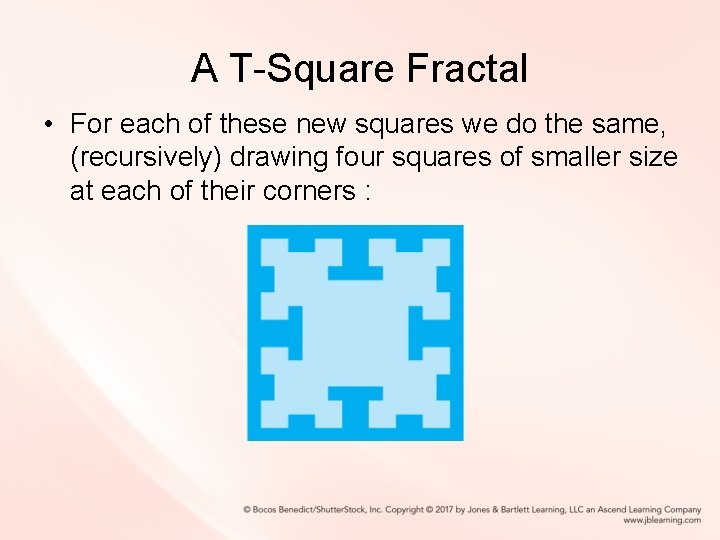A T-Square Fractal • For each of these new squares we do the same, (recursively) drawing four squares of smaller size at each of their corners :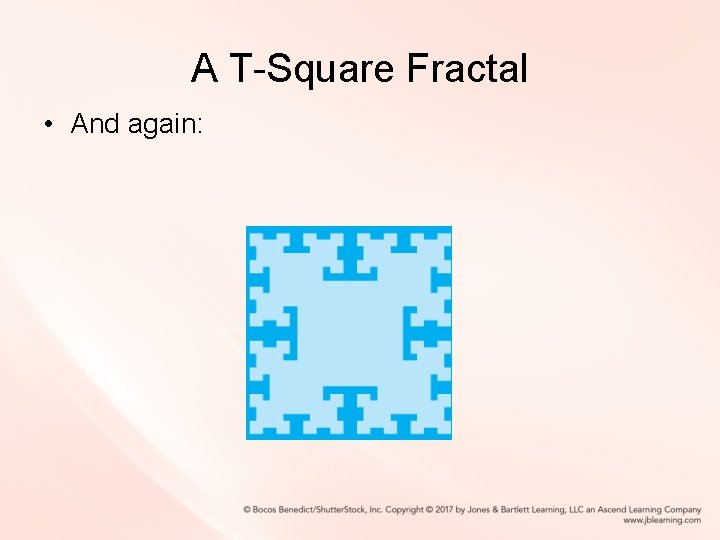A T-Square Fractal • And again:A T-Square Fractal • And again: until we can no longer draw any more squares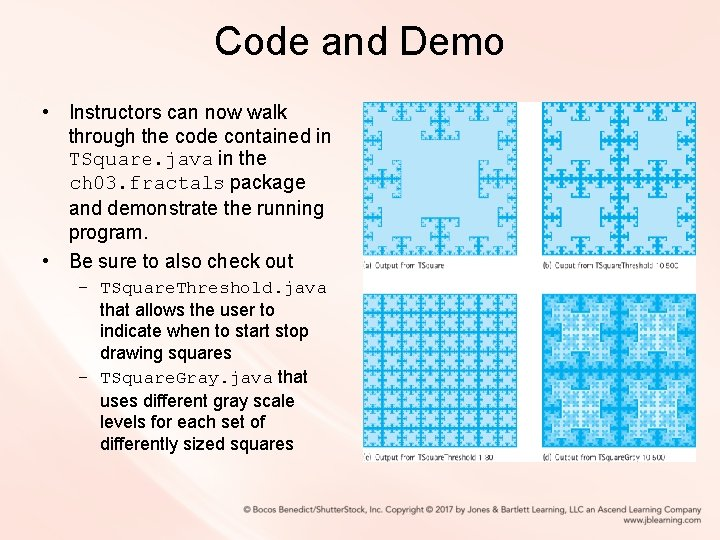Code and Demo • Instructors can now walk through the code contained in TSquare. java in the ch 03. fractals package and demonstrate the running program. • Be sure to also check out – TSquare. Threshold. java that allows the user to indicate when to start stop drawing squares – TSquare. Gray. java that uses different gray scale levels for each set of differently sized squares3. 7 Removing Recursion • We consider two general techniques that are often substituted for recursion – iteration – stacking. • First we take a look at how recursion is implemented. – Understanding how recursion works helps us see how to develop non-recursive solutions.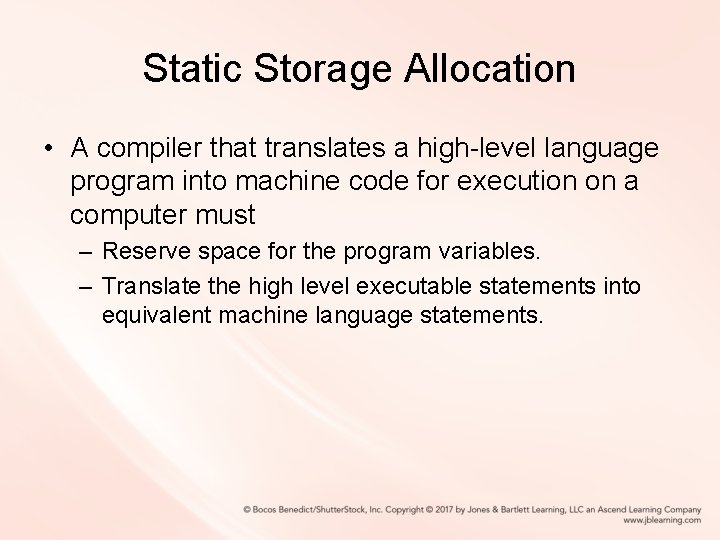Static Storage Allocation • A compiler that translates a high-level language program into machine code for execution on a computer must – Reserve space for the program variables. – Translate the high level executable statements into equivalent machine language statements.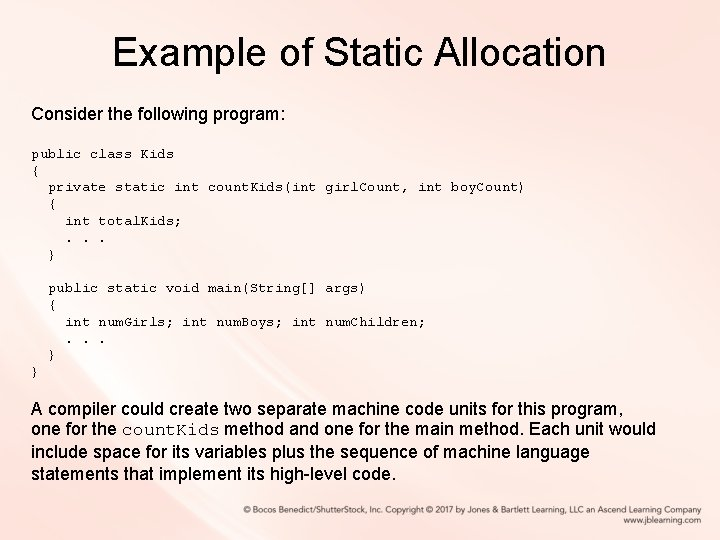Example of Static Allocation Consider the following program: public class Kids { private static int count. Kids(int girl. Count, int boy. Count) { int total. Kids; . . . } public static void main(String[] args) { int num. Girls; int num. Boys; int num. Children; . . . } } A compiler could create two separate machine code units for this program, one for the count. Kids method and one for the main method. Each unit would include space for its variables plus the sequence of machine language statements that implement its high-level code.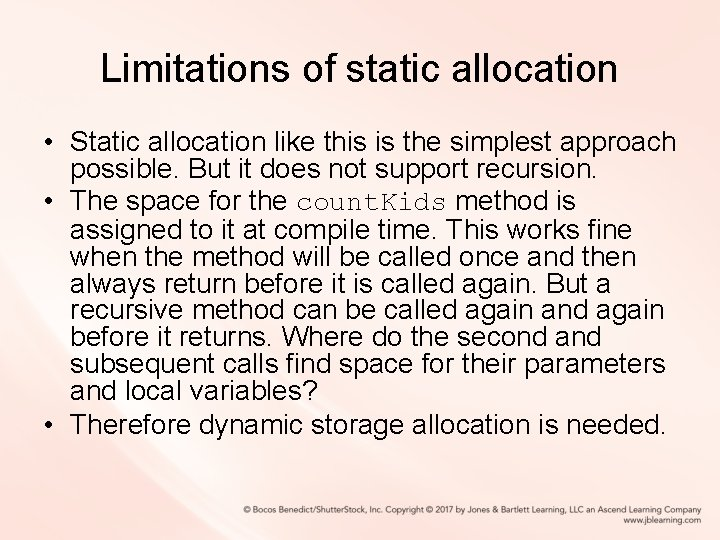Limitations of static allocation • Static allocation like this is the simplest approach possible. But it does not support recursion. • The space for the count. Kids method is assigned to it at compile time. This works fine when the method will be called once and then always return before it is called again. But a recursive method can be called again and again before it returns. Where do the second and subsequent calls find space for their parameters and local variables? • Therefore dynamic storage allocation is needed.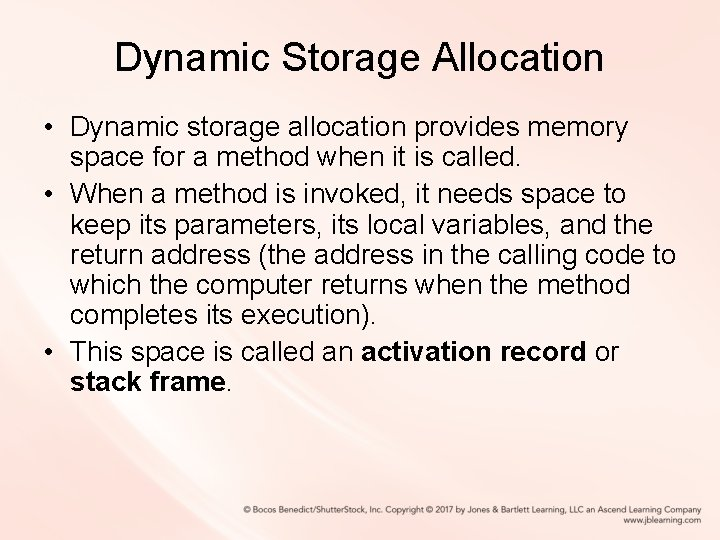Dynamic Storage Allocation • Dynamic storage allocation provides memory space for a method when it is called. • When a method is invoked, it needs space to keep its parameters, its local variables, and the return address (the address in the calling code to which the computer returns when the method completes its execution). • This space is called an activation record or stack frame.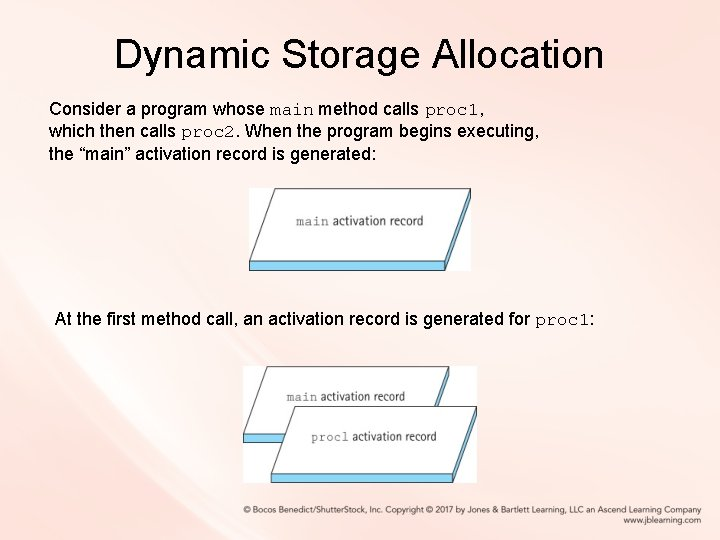Dynamic Storage Allocation Consider a program whose main method calls proc 1, which then calls proc 2. When the program begins executing, the “main” activation record is generated: At the first method call, an activation record is generated for proc 1:Dynamic Storage Allocation When proc 2 is called from within proc 1, its activation record is generated. Because proc 1 has not finished executing, its activation record is still around: When proc 2 finishes executing, its activation record is released: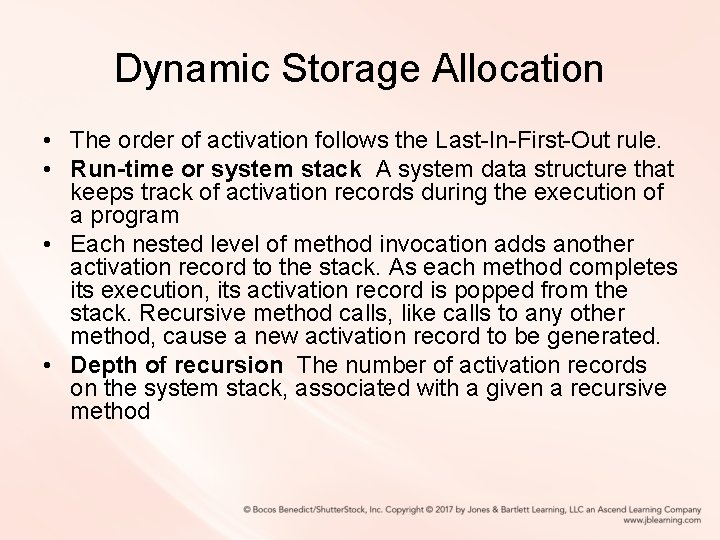Dynamic Storage Allocation • The order of activation follows the Last-In-First-Out rule. • Run-time or system stack A system data structure that keeps track of activation records during the execution of a program • Each nested level of method invocation adds another activation record to the stack. As each method completes its execution, its activation record is popped from the stack. Recursive method calls, like calls to any other method, cause a new activation record to be generated. • Depth of recursion The number of activation records on the system stack, associated with a given a recursive method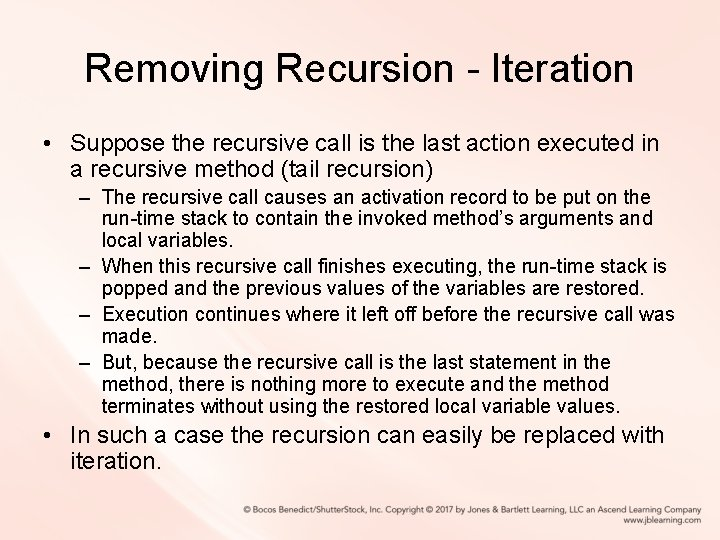Removing Recursion - Iteration • Suppose the recursive call is the last action executed in a recursive method (tail recursion) – The recursive call causes an activation record to be put on the run-time stack to contain the invoked method’s arguments and local variables. – When this recursive call finishes executing, the run-time stack is popped and the previous values of the variables are restored. – Execution continues where it left off before the recursive call was made. – But, because the recursive call is the last statement in the method, there is nothing more to execute and the method terminates without using the restored local variable values. • In such a case the recursion can easily be replaced with iteration.Example of eliminating tail recursion public static int factorial(int n) { if (n == 0) return 1; // Base case else return (n * factorial(n – 1)); } Declare a variable to hold the intermediate values; initialize it to the value returned in the base case. Use a while loop so that each time through the loop corresponds to one recursive call. The loop should continue processing until the base case is met: // General case private static int factorial(int n) { int ret. Value = 1; // return value while (n != 0) { ret. Value = ret. Value * n; n = n - 1; } return(ret. Value); }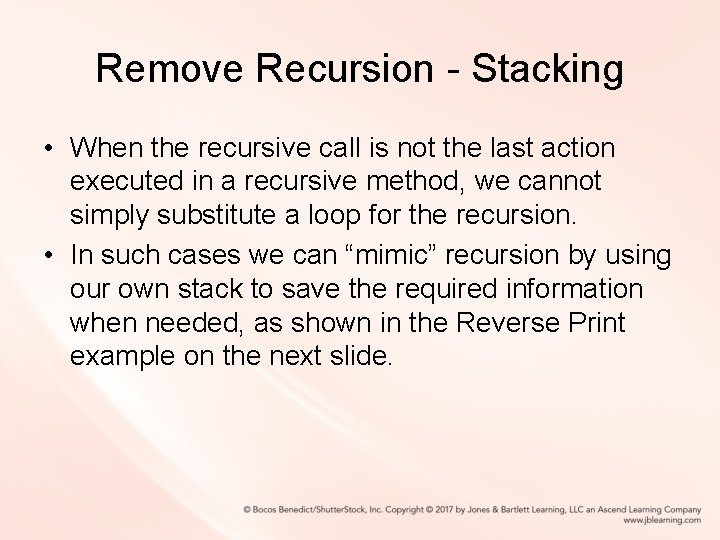Remove Recursion - Stacking • When the recursive call is not the last action executed in a recursive method, we cannot simply substitute a loop for the recursion. • In such cases we can “mimic” recursion by using our own stack to save the required information when needed, as shown in the Reverse Print example on the next slide.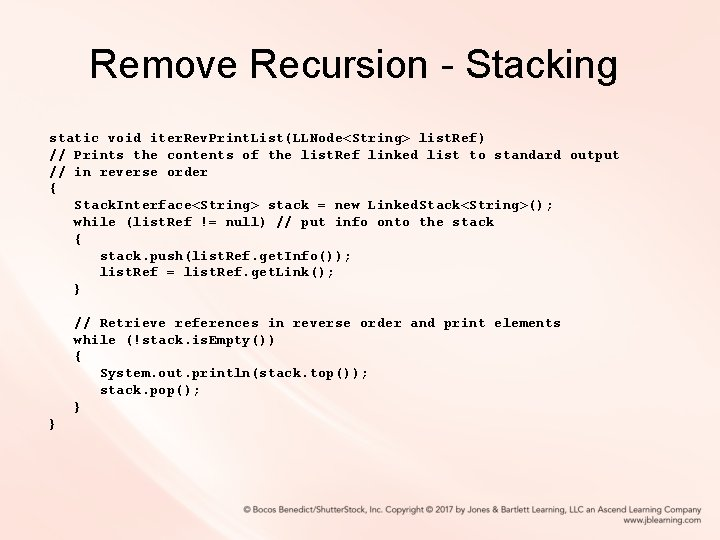Remove Recursion - Stacking static void iter. Rev. Print. List(LLNode<String> list. Ref) // Prints the contents of the list. Ref linked list to standard output // in reverse order { Stack. Interface<String> stack = new Linked. Stack<String>(); while (list. Ref != null) // put info onto the stack { stack. push(list. Ref. get. Info()); list. Ref = list. Ref. get. Link(); } // Retrieve references in reverse order and print elements while (!stack. is. Empty()) { System. out. println(stack. top()); stack. pop(); } }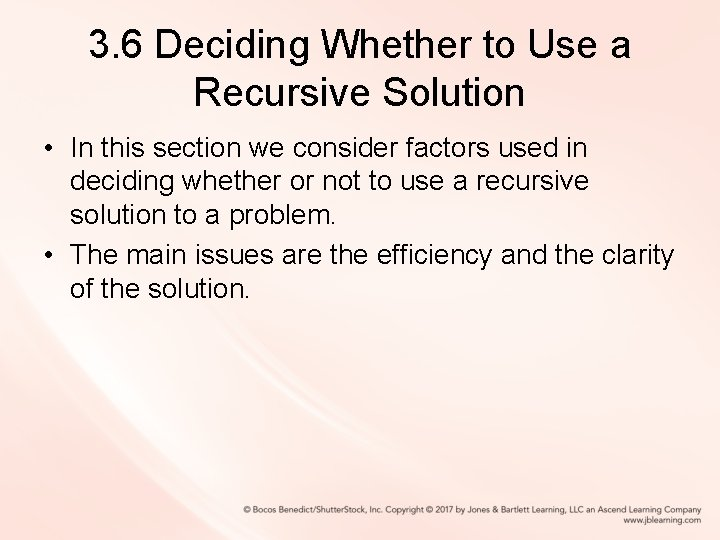3. 6 Deciding Whether to Use a Recursive Solution • In this section we consider factors used in deciding whether or not to use a recursive solution to a problem. • The main issues are the efficiency and the clarity of the solution.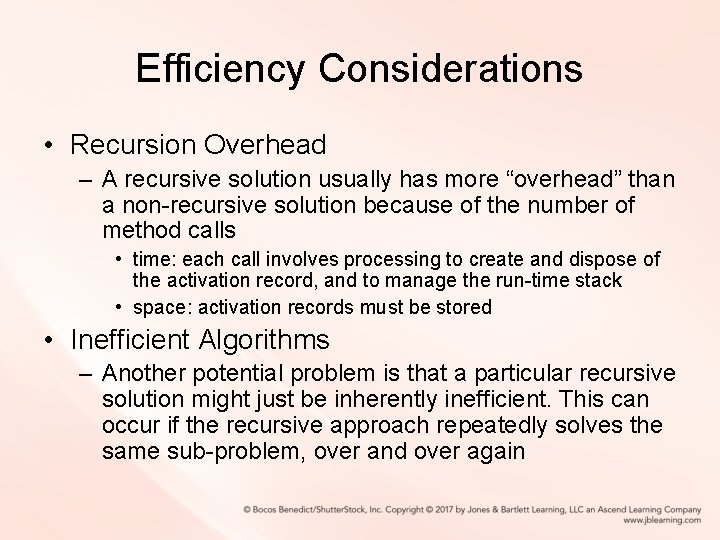Efficiency Considerations • Recursion Overhead – A recursive solution usually has more “overhead” than a non-recursive solution because of the number of method calls • time: each call involves processing to create and dispose of the activation record, and to manage the run-time stack • space: activation records must be stored • Inefficient Algorithms – Another potential problem is that a particular recursive solution might just be inherently inefficient. This can occur if the recursive approach repeatedly solves the same sub-problem, over and over again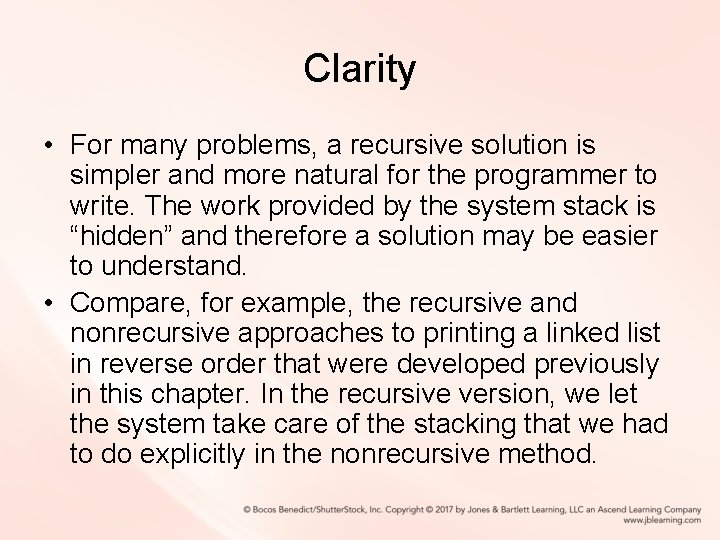Clarity • For many problems, a recursive solution is simpler and more natural for the programmer to write. The work provided by the system stack is “hidden” and therefore a solution may be easier to understand. • Compare, for example, the recursive and nonrecursive approaches to printing a linked list in reverse order that were developed previously in this chapter. In the recursive version, we let the system take care of the stacking that we had to do explicitly in the nonrecursive method.